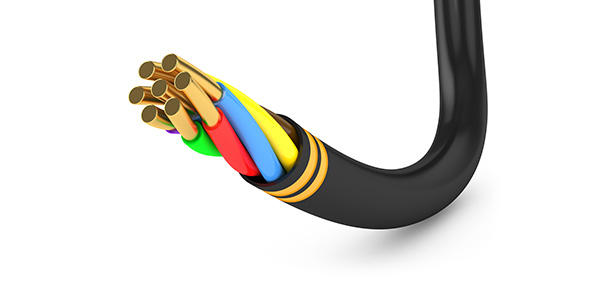# Electrical Definition Exam Quiz

30 Questions | Total Attempts: 363Settings• 1.
- The SI unit of electric charge equal to the quantity of electricity     transferred      along the conductor by a current of one ampere in one second
• A.

KILOWATT (kW)

• B.

COULUMB (C)

• C.

WATTAGE

• D.

POTENTIAL DIFFERENCE

• 2.
The energy per unit charge available for conversion from a chemical,          mechanical or other form of energy into electrical energy or vice versa in a     conversion devise as a battery, generator or a motor
• A.

ELECTROMOTIVE FORCE (emf)

• B.

VOLTAGE

• C.

AMPERE (A)

• D.

OHM

• 3.
The basic SI unit of electric current, equivalent to a flow of one coulomb     per       second or to the steady current produced by one volt applied to one   resistance of   one ohm
• A.

AMPERAGE

• B.

CIRCUIT

• C.

AMPERE (A)

• D.

PARALLEL

• 4.
The SI unit equal to one joule per second or to the power represented by     a          current of one ampere flowing across a potential difference of one volt             W= V x A
• A.

WATT (W)

• B.

ELECTROMOTIVE FORCE (emf)

• C.

POTENTIAL DIFFERENCE

• D.

RESISTANCE (R)

• 5.
The strength of an electric current measured or expressed in amperes:       analogous to the rate of water flow
• A.

AMPERAGE

• B.

RESISTANCE (R)

• C.

BATTERY

• D.

ELECTRODE

• 6.
A pliable metallic strand or twisted or woven assembly of such strands        often    insulated with a dielectric material and used as a conductor of    electricity
• A.

INSULATOR

• B.

WIRE

• C.

NON- METALLIC SHEATED CABLE

• D.

SHIELDED CABLE

• 7.
An electric cable enclosed within a metallic sheath in order to reduce the    effects of external electric or magnetic fields
• A.

COAXIAL CABLE

• B.

FLEXIBLE METAL CONDUIT

• C.

RIGID METAL CONDUIT

• D.

SHIELDED CABLE

• 8.
The minimum applied voltage at which a given insulator breaks down and   permits current to pass
• A.

BREAKDOWN VOLTAGE

• B.

DIELECTRIC

• C.

JUNCTION BOX

• D.

GROMMET

• 9.
An enclosure for housing and protecting electric wires or cables that are     joined together in connecting or branching electric circuits
• A.

PULL BOX

• B.

THWN

• C.

INSULATOR

• D.

JUNCTION BOX

• 10.
An amount of power especially the power required to operate an     electrical          device or appliance, expressed in watts
• A.

WATTAGE

• B.

KILOWATT (kW)

• C.

COULUMB (C)

• D.

WATT (W)

• 11.
A unit of power equal to 1,000 watts
• A.

KILOWATT-HOUR (kWh)

• B.

POTENTIAL DIFFERENCE

• C.

VOLTAGE

• D.

KILOWATT (kW)

• 12.
Potential difference or electromotive force expressed in volts: analogous    to         pressure in water flow
• A.

VOLT (V)

• B.

CURRENT

• C.

VOLTAGE

• D.

AMPERE (A)

• 13.
The opposition of a conductor to the flow of current, causing some of the    electric            energy to be transformed into heat and usually measured in ohms
• A.

AMPERE (A)

• B.

AMPERAGE

• C.

OHM

• D.

RESISTANCE (R)

• 14.
The principle that the rate of production of heat by direct current is directly proportional to the resistance of the circuit and to the square of the current
• A.

CIRCUIT

• B.

CONDUCTIVITY

• C.

RESISTIVITY

• D.

JOULE’S LAW

• 15.
A measure of the ability of a substance to conduct electric current, equal    to the reciprocal of the resistivity of the substance. Also called specific           conductance.
• A.

CONDUCTIVITY

• B.

SERIES

• C.

PARALLEL

• D.

BATTERY

• 16.
RHW
• A.

Moisture resistant thermoplastic

• B.

Moisture and heat resistance rubber

• C.

Asbestos and varnished cambric

• D.

Silicone asbestos

• 17.
XHHW
• A.

Silicone asbestos

• B.

Heat resistant thermoplastic

• C.

Moisture & heat resistant thermoplastic

• D.

Moisture & heat resistant cross linked

• 18.
THW
• A.

Moisture & heat resistant thermoplastic

• B.

Moisture resistant thermoplastic

• C.

Moisture and heat resistance rubber

• D.

Asbestos and varnished cambric

• 19.
Used as supports and for additional protection for wires
• A.

CUT OUT BOX

• B.

INSULATOR

• C.

OUTLET BOX

• D.

BUSHING

• 20.
Where cable ends
• A.

UTILITY BOX

• B.

PULL BOX

• C.

CUT OUT BOX

• D.

OUTLET BOX

• 21.
Metal box with hinge & enclosure. House or fuse box
• A.

CUT OUT BOX

• B.

OUTLET BOX

• C.

PULL BOX

• D.

UTILITY BOX

• 22.
A rubber or plastic washer inserted in a hole in a metal part to prevent         grounding of a wire passing through the hole
• A.

KNOCKOUT

• B.

GROMMET

• C.

JUNCTION BOX

• D.

BUSHING

• 23.
The maximum voltage that can be applied to a given material without          causing it to breakdown, usually expressed in volts or kilovolts per unit of        thickness
• A.

DIELECTRIC STRENGTH

• B.

DIELECTRIC

• C.

BREAKDOWN VOLTAGE

• D.

CABLE TRAY

• 24.
An open metal framework for supporting insulated electrical conductors
• A.

BUS DUCT

• B.

DUCT

• C.

MULTI- OUTLET ASSEMBLY

• D.

CABLE TRAY

• 25.
A surface mounted raceway designed to house the electrical wires for a     circuit and a series of receptacles
• A.

MULTI- OUTLET ASSEMBLY

• B.

SURFACE RACEWAY

• C.

RACEWAY

• D.

FLEXIBLE METAL CONDUIT

Related TopicsBack to top